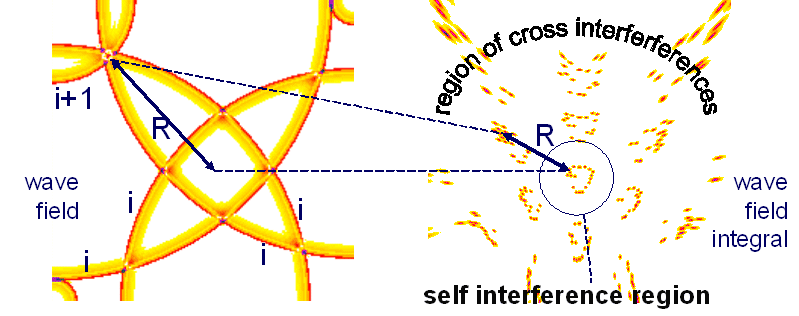14th Herbstakademie THEORY IN COGNITIVE NEUROSCIENCE November 4th - 7th, 2007 in Wildbad Kreuth, Bavaria, Germany organized by Harald Atmanspacher and Wolfgang Tschacher Conference website: http://www.upd.unibe.ch/research/symposien/HA14.html Nerve Velocity Calculated by Cross Interference Distance Gerd Heinz, GFaI Berlin info@gheinz.de, www.gheinz.de Abstract Signals in nerve systems can be seen as pulse-like time-functions, flowing slowly through different stages of information processing. We will call them discrete waves on wires that flow in inhomogeneous nets of wires, so called "Interference Networks" (IN). Excitement locations (interference integrals) are coupled to places, were many wave maxima interfere. Different pioneers found different views on such nets between theoretical and experimental sciences. Karl Lashley analysed holographic properties at rats (1920-1950), Lloyd A. Jeffress (1948) and Mark Konishi (1993) discussed first interference circuits, Karl Pribram asked for holomorphic relations, Walter Freeman gave 1972 the first wave impression and Andrew Packard observed 1995 waves on animals (octopus). Introducing the terminus 'time-function wave' the author observed some general relations and properties of interference nets between wave fields and wave field integrals ('images') since 1992. Basic properties of IN were investigated with simple, for demonstration homogeneous configurations. Data addressing in IN needs the self-interference condition, like in optical images, projections can only occur under certain circumstances at defined places, at locations of meeting of pulses (index i) of the same time origin (called self-interference). But if any (pulse-) wave i has following or foregoing pulses with wave indizes i+1, i-1, i+2,..., i+n, i-n interferences occure also between them (called 'permutations' in Neuronale Interferenzen, 1993). Such interferences between subsequent waves of different channels produce figures around the self-interference region, called cross-interference figures, see right figure. If subsequent pulses flow with specific velocity, the pulse-pause corresponds to a geometric distance, the geometric wave length. If many pulses flow through different nerves and re-combine, a specific pattern shows the so called cross-interference distance: Around a self-interference figure subsequent pulses form a cross-interference pattern, which has a distance to the self-interference figures. To address data one-to-one without cross-interference disturbances, the space has to be free of cross interferences - only the region of self-interference can be used. If not, we get ambiguities in the interaction between cortex and sensoric or motoric places. If it is possible, to measure the distance between the self- and the cross-interference region, it would be possible to give a measure for general properties of such nets.However, the average distance between self- and cross interference pattern has its reason in the average pulse-pause between the waves. So the cross-interference distance R appears as the average distance between waves (1) R = vT/2 with average nerve velocity v and pulse distance (pause) T = 1/f, fire frequency is f. See also (Eqn. (1) in Mathematik des Nervensystems. If we change the view, we can ask for the required velocity v for a cross interference distance R of two meter for a long Swiss Guardian. Supposed, he will locate all sensory locations on his body correctly, R has to get the size of the self-interference region. We find (2) v = 2 R/T = 2 f R. Example: For a body of two meter length R and a maximum fire rate f of 30 Hz we get a velocity v R = 2 m; f = 30 Hz v = 2 f R = 2* 30 Hz * 2 m = 120 m/s to hold the interference system within the self-interference region. Dependent of the firing rate and of geometric configurations we get a value for the velocity v of 120 m/s. The value corresponds to the velocity measurable in our myelin-isolated nerve system. This seems not to be a coincidence only. It shows the possibility to calculate exciting properties of peripheral nerve system using Interference Networks.